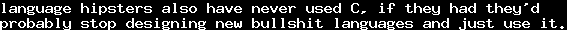# Equality of computable functions from boolean sequences to natural numbers is decidableSun, 17 Apr 2011 13:00:00 GMT

I was just told that the equality of computable functions of typeis decidable. I was a bit confused about this at first, since trivially, the equality of functionsis undecidable: Letbe the a complete enumeration of all proofs in our meta-theory, then define, then by Gödel's second incompleteness theorem, we cannot decide whether.

However, even though the question whether two computable functionsare equal seems harder than the same question for, it is not. A first step in understanding why is maybe that every terminating algorithm is finite, and therefore can only use finitely many elements of a sequence in.

Of course, having this information without any proofs I tried to prove it myself. I noticed that this should be strongly related to the compactness theorem. And here is the outcome:

Lemma 1:
Let a computablebe given, and. Then there is some initial subsequenceof, such that for all sequences,.
Proof: A terminating turing machine can, for every given sequence, only read finitely many elements of, thus, there is an upper boundsuch that the program is only dependent of elements. Setand. Thenfor all sequences, since the program does not depend on an elements not in. ‏
□‎

We say that a computabledepends only on a sequencewhen for everywe have, and it depends exactly onwhen it depends only onbut this is not the case for subsequences.

By Lemma 1 it is trivial to proof that two functionsare not equal: Just find a sequenceon that both of them depend only, and for which. The more interesting part is the case when they are equal.

Using Lemma 1, we can encode such functionsin the following way: Letbe predicate constants, and denote bythe first-order Peano arithmetic. Ifwheredepends only on, then let, whereis a term constant, andcan be expressed in the formas usual. Now define. Obviously,. Now, if two functionsare equal, we know thatis not satisfiable, and therefore,. By the completeness theorem, we therefore know that, and hence, the equalityis provable.

Nice.